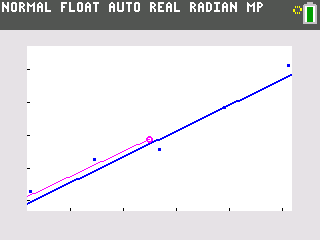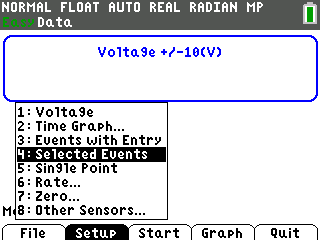# Activities

••• ##### Subject Area

• Math: Algebra I: Linear Functions
• Science: Physics: Electrostatics

• ##### Author9-12

60 Minutes

• ##### Device
• TI-83 Plus Family
• TI-84 Plus
• TI-84 Plus Silver Edition
•TI-84 Plus C Silver Edition
•TI-84 Plus CE
• ##### Software

TI Connect™
TI Connect™ CE

• ##### Accessories

Sensor - Voltage
TI Connectivity Cable

• ##### Other Materials
This is Activity 9 from the EXPLORATIONS Book:
EasyData Activities: Modeling Algebraic Functions with Data Collection Activities.

The following materials are required for this activity:
• 5 batteries of same size and 1.5 volts
• Battery holder or ruler
• ##### Report an Issue#### Activity Overview

Students explore the total voltage provided by several batteries in a series to a battery-operated device. They graph scatter plots, understand multiplication as repeated addition, and use a pattern to develop a formula. They learn how to develop a formula from a pattern of increasing voltage and graph increase in voltage in the form of a scatter plot.

#### Key Steps

•Using a Voltage Sensor and a Vernier EasyLinkTM students place a set of batteries in series and calculate the total voltage.

•The data is then graphed and the data is modeled with both a regression equation and a manually fit line. Students then compare their line to the regression equation.